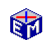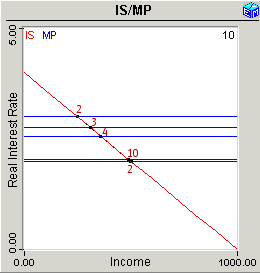The IS/MP Model

The IS/MP Model replaces the LM curve in the IS/LM Model with a monetary policy (MP) curve and changes the vertical axis from the nominal interest rate to the real interest rate.  These changes allow the IS/MP Model to focus on monetary policy in terms of the rate of inflation instead of the price level.  (See the references below for a complete description of the model.)

The IS/MP Model application first explains the elements that are new.  These are:

• Monetary Policy (MP) Curve

You can then run either fiscal policy or monetary policy experiments.  The simulations immediately show you the short run effects.  You move forward through time to trace out the long run effects.

The diagrams below are an example of this analysis.Model LinkThe IS/MP Model
Printable PDF Exercises

The IS/MP Model is fairly recent.  The structure is described in David Romer's paper Short-Run Fluctuations, which is available below.  (You will need both documents.)

Paper    Tables

The IS/MP Model presented here follows closely the details in "Keynesian Macroeconomics without the LM Curve," by David Romer in the Journal of Economic Perspectives (Spring, 2000).These diagrams show the dynamic path the economy follows when the Fed moves to increase the real interest rate in period 2.  By period 10, the economy has returned to nearly the original level of income.  These diagrams are screen shots from the EconModel presentation of the IS/MP Model.

Classic Economic Models

Macroeconomics

Introduction
Overview of Macro Models

Microeconomics

Introduction
Overview of Micro Models

Resources